Courses

# Theory & Procedure, Metre Bridge (Resistance of a wire) Class 12 Notes | EduRev

## JEE : Theory & Procedure, Metre Bridge (Resistance of a wire) Class 12 Notes | EduRev

The document Theory & Procedure, Metre Bridge (Resistance of a wire) Class 12 Notes | EduRev is a part of the JEE Course Physics Class 12.
All you need of JEE at this link: JEE

Our Objective:

To find the resistance of a given wire using a metre bridge and hence determine the specific resistance of its materials.

Theory

Wheatstone’s principle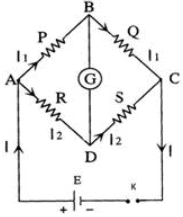The metre bridge is operates under Wheatstone’s principle.  Here, four resistors P, Q, R, and S are connected to form the network ABCD. The terminals A and C are connected to a battery, and the terminals C and D are connected to a galvanometer through keys K1 and K2 respectively.
In the balancing condition, there is no deflection on the galvanometer. Then,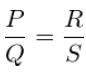Metre Bridge apparatus

The metre bridge, also known as the slide wire bridge consists of a one metre long wire of uniform cross sectional area, fixed on a wooden block. A scale is attached to the block. Two gaps are formed on it by using thick metal strips in order to make the Wheat stone’s bridge. The terminal B between the gaps is used to connect galvanometer and jockey.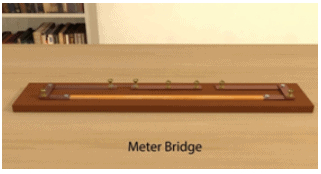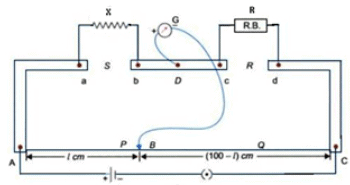A resistance wire is introduced in gap S and the resistance box is in gap R. One end of the galvanometer is connected to terminal D and its other end is connected to a jockey. As the jockey slides over the wire AC, it shows zero deflection at the balancing point (null point).

If the length AB is, then the length BC is  ( 100-l ).

Then, according to Wheatstone’s principle;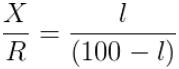Now, the unknown resistance can be calculated as,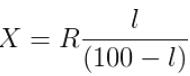The specific resistance or resistivity of the material of the wire can be then calculated by using the relation,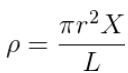; Where L be the length of the wire and r be its radius.

Learning Outcomes:

• Students understand Wheatstone’s bridge and Wheatstone’s principle.
• Students verify Wheatstone’s principle.
• Students correlate the principle of Wheatstone’s bridge with metre bridge experiment.

Materials required: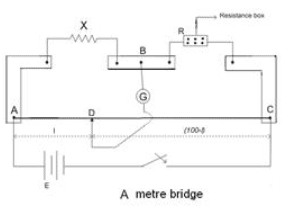• Metre bridge (slide wire bridge)
• Leclanche cell or Battery eliminator
• Galvanometer
• Resistance box
• Jockey
• One way key
• A resistance wire
• Screw gauge
• Metre scale
• Connecting wires

Real Lab Procedure:

• Arrange the required materials on a table and make the connections as per the connections diagram.
• Connect the resistance wire in the left gap (between c & d) and resistance box in the right gap.
• Introduce some resistance in the circuit by taking out some resistance from the resistance box.
• Plug the key. Bring the jockey in contact with the end A first, and then with C.  Note the deflection on the galvanometer.
• If the galvanometer deflects in the opposite direction, the connections are right and the null point is in between A and C.
• If not so, change the resistance in the resistance box and repeat the process so that the null point is somewhere between A and C.
• If the galvanometer deflected towards a single side, then check the connection.
• Now, slide the jockey slowly over the wire starting from one and (say, A) and note the galvanometer deflection. Continue the process till the balancing point is reached.
• Balancing point is the point at which the galvanometer shows zero deflection. Now, note the position of the jockey from end A. Take it as the balancing length (l) using the metre scale.
• Repeat the process for different values of R. The balancing length is measured  each time.
• Now, interchange the position of resistance wire and resistance box in gaps AB and CD.
• Repeat the above steps to find the balancing length, for the same values of R.
Offer running on EduRev: Apply code STAYHOME200 to get INR 200 off on our premium plan EduRev Infinity!

## Physics Class 12

204 videos|288 docs|125 tests

,

,

,

,

,

,

,

,

,

,

,

,

,

,

,

,

,

,

,

,

,

,

,

,

;